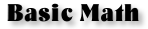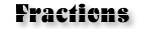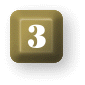## Interactive Math QuizzesAll questions have answers lower than 100. Addition, subtraction and multiplication.New! Addition, Subtraction, Multiplication and Division... all options available on our new Fraction Quiz.All answers will be below 1,000. There is an option to allow negative numbers for answers to Subtraction questions. Addition, subtraction and multiplication.Division and multiplication of fractions has now been combined with Addition and subtraction options.All answers will be between 0 and 10,000. Addition, subtraction and multiplication.Math and Money... Percentages, Interest, Commissions, Profit and Loss, Mortgages, Credit Cards and Compound Interest Calculator.